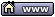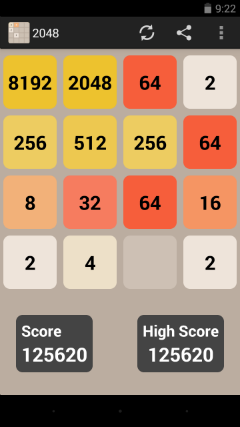flat assembler
Message board for the users of flat assembler.flat assembler > Heap > 2048 Goto page Previous  1, 2
Author
 Threadrevolution
When all else fails, read the source

Joined: 24 Aug 2004
Posts: 16462
Location: Crab Nebula
Tyler wrote:
Revolution, does a screenshot with a previous highscore (the number in the bottom right) count?
No. I saw posted the block value of 131072 (2^17).16 Aug 2014, 07:12sleepsleep

Joined: 05 Oct 2006
Posts: 8071
Location: ˛　　　　　　　　　　　　　　　　　　　　　　　　　　　　　　⁣⁣⁣⁣⁣⁣⁣⁣⁣⁣⁣⁣⁣⁣⁣⁣⁣⁣⁣⁣⁣⁣⁣⁣⁣⁣⁣⁣⁣⁣⁣⁣⁣⁣⁣⁣⁣⁣⁣⁣⁣⁣⁣⁣ Posts: 6699
after a few months, i got 125620 score =)
i should get higher scores if not few stupid mistakes....

 Description: Filesize: 21.31 KB Viewed: 1648 Time(s)28 Mar 2015, 14:26sleepsleep

Joined: 05 Oct 2006
Posts: 8071
Location: ˛　　　　　　　　　　　　　　　　　　　　　　　　　　　　　　⁣⁣⁣⁣⁣⁣⁣⁣⁣⁣⁣⁣⁣⁣⁣⁣⁣⁣⁣⁣⁣⁣⁣⁣⁣⁣⁣⁣⁣⁣⁣⁣⁣⁣⁣⁣⁣⁣⁣⁣⁣⁣⁣⁣ Posts: 6699
updating my new score =)21 Apr 2015, 20:48Picnic

Joined: 05 May 2007
Posts: 1271
Location: Microlimano
Nice puzzle. I have not yet managed to reach 2048 but I am close, i just need more practiceHere is a version for my toy interpreter.
Code:
```
rem  2048 Puzzle Game
rem  Use the arrow keys to move the tiles
rem  When two tiles with the same number touch, they merge into one!

VK_UP=38
VK_DOWN=40
VK_LEFT=37
VK_RIGHT=39

dim board[4,4]
dim blocked[4,4]

path\$=PATH(1)+"\ART\2048.TXT"
if SIZE(path\$)=-1 then file path\$,"0",0
file path\$,best\$,1
best\$=TRIM(best\$)
delay=10000

title "2048 Game in Hobby Basic"
screen 80,30
cursor 0,0
pen 3
at 63,4,"SCORE:"
at 63,6,"BEST:"
pen 7
at 0,22,"Join the numbers and get to the 2048 tile!"
at 0,23,"Use the arrow keys to move the tiles."
at 0,24,"Press R to restart, Esc to quit."

start#

done=0
score=0
moved=1
erase 70,4,7,-1,-1
erase 70,6,7,-1,-1
erase 0,25,80,-1,-1
erase 0,26,80,-1,-1

for y=0 to 3
for x=0 to 3
board[x,y]=0
blocked[x,y]=0
next x
next y

do
if moved then addTile()
drawBoard()
if done then break
waitKey()
until done

if score>VAL(best\$)
best\$=STR(score)
file path\$,best\$,0
endif

if done=27 then end
if done='R' then done=0:goto start
locate 0,25:? "No more moves."
if isWinner() then ? "You''ve made it!" else ? "You lose!"
@@# inkey:k=V0&0x5F
if k='R' then goto start
if k<>27 then goto @b
end

sub drawBoard()

local x,y

at 70,4,score
at 70,6,best\$

for y=0 to 3
for x=0 to 3
v=board[x,y]:if v=0 then i=5 else i=getColor(v)
color i,0:paint 34+(x*7),3+(y*4),6,3,0x20,1
if i<>5 then at 34+(x*7),4+(y*4),PAD(STR(v),0x20,5)
next x
next y
color 0,7
ends

sub waitKey()

local x,y,k

moved=0
inkey:k=V0&0x5F
if (k=27 or k='R') then done=k:rets
k=V2:if (k>36 and k<41) then movDir(k)
for y=0 to 3
for x=0 to 3
blocked[x,y]=0
next x
next y
ends

local x,y,a,b

for y=0 to 3
for x=0 to 3
if ~board[x,y]
do:a=RND(4):b=RND(4):until ~board[a,b]
if RND(100)>89 then board[a,b]=4 else board[a,b]=2
if canMove() then rets
endif
next x
next y

done=1
ends

sub canMove()

local x,y

for y=0 to 3
for x=0 to 3
if ~board[x,y] then rets 1
next x
next y

for y=0 to 3
for x=0 to 3
if testAdd(x+1,y,board[x,y]) then rets 1
if testAdd(x-1,y,board[x,y]) then rets 1
if testAdd(x,y+1,board[x,y]) then rets 1
if testAdd(x,y-1,board[x,y]) then rets 1
next x
next y

rets 0
ends

if (x<0 or x>3 or y<0 or y>3) then rets 0
if board[x,y]=v then rets 1

rets 0
ends

sub moveVert(x,y,d)

if (board[x,y+d] and board[x,y+d]=board[x,y] and ~blocked[x,y] and ~blocked[x,y+d])
board[x,y]=0
board[x,y+d]=board[x,y+d]*2
score=score+board[x,y+d]
blocked[x,y+d]=1
moved=1
elseif (~board[x,y+d] and board[x,y])
board[x,y+d]=board[x,y]
board[x,y]=0
moved=1
endif

if d>0
if y+d<3 then drawBoard():wait delay:moveVert(x,y+d,1)
else
if y+d>0 then drawBoard():wait delay:moveVert(x,y+d,-1)
endif
ends

sub moveHori(x,y,d)

if (board[x+d,y] and board[x+d,y]=board[x,y] and ~blocked[x,y] and ~blocked[x+d,y])
board[x,y]=0
board[x+d,y]=board[x+d,y]*2
score=score+board[x+d,y]
blocked[x+d,y]=1
moved=1
elseif (~board[x+d,y] and board[x,y])
board[x+d,y]=board[x,y]
board[x,y]=0
moved=1
endif

if d>0
if x+d<3 then drawBoard():wait delay:moveHori(x+d,y,1)
else
if x+d>0 then drawBoard():wait delay:moveHori(x+d,y,-1)
endif
ends

sub movDir(d)

local x,y

if d=VK_UP
for x=0 to 3
y=1
while y<4
if board[x,y] then moveVert(x,y,-1)
y=y+1
endw
next x
elseif d=VK_DOWN
for x=0 to 3
y=2
while y>=0
if board[x,y] then moveVert(x,y,1)
y=y-1
endw
next x
elseif d=VK_LEFT
for y=0 to 3
x=1
while x<4
if board[x,y] then moveHori(x,y,-1)
x=x+1
endw
next y
elseif d=VK_RIGHT
for y=0 to 3
x=2
while x>=0
if board[x,y] then moveHori(x,y,1)
x=x-1
endw
next y
endif
ends

sub isWinner()

for y=0 to 3
for x=0 to 3
if board[x,y] >= 2048 then rets 1
next x
next y

rets 0
ends

sub getColor(c)

i=0
while c>2
c=c>>1
i=i+1:if i>6 then i=0
endw

rets i+9
ends
```Last edited by Picnic on 25 Mar 2019, 13:49; edited 7 times in total06 Feb 2018, 19:52sleepsleep

Joined: 05 Oct 2006
Posts: 8071
Location: ˛　　　　　　　　　　　　　　　　　　　　　　　　　　　　　　⁣⁣⁣⁣⁣⁣⁣⁣⁣⁣⁣⁣⁣⁣⁣⁣⁣⁣⁣⁣⁣⁣⁣⁣⁣⁣⁣⁣⁣⁣⁣⁣⁣⁣⁣⁣⁣⁣⁣⁣⁣⁣⁣⁣ Posts: 6699
hi Picnic,
somehow this game doesnt made into my new phone,

nice syntax,08 Feb 2018, 18:59Display posts from previous: All Posts1 Day7 Days2 Weeks1 Month3 Months6 Months1 Year Oldest FirstNewest FirstJump to: Select a forum Official----------------AssemblyPeripheria General----------------MainDOSWindowsLinuxUnixMenuetOS Specific----------------MacroinstructionsCompiler InternalsIDE DevelopmentOS ConstructionNon-x86 architecturesHigh Level LanguagesProgramming Language DesignProjects and IdeasExamples and Tutorials Other----------------FeedbackHeapTest Area
Goto page Previous  1, 2

Forum Rules:
 You cannot post new topics in this forumYou cannot reply to topics in this forumYou cannot edit your posts in this forumYou cannot delete your posts in this forumYou cannot vote in polls in this forumYou can attach files in this forumYou can download files in this forum